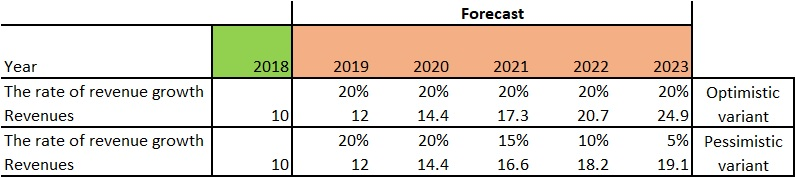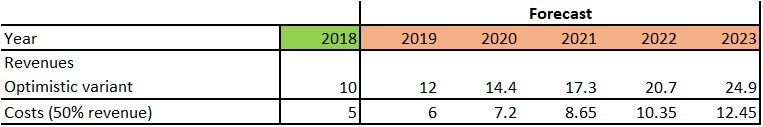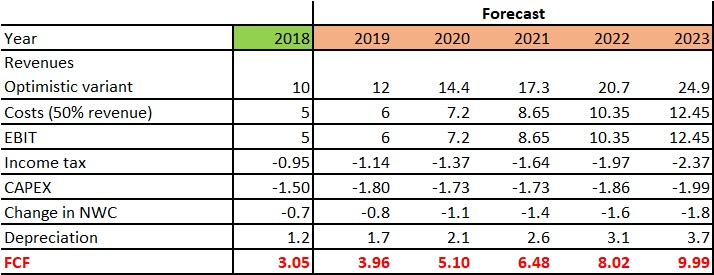In the second part of the article on a valuation of a company using the Income Approach (first part here) we will discuss the most popular variant of it, namely Free Cash Flow to Firm. In this model, we must determine future cash flows for the entire company, and then bring them back to the point where we make the valuation, which is to discount the free cash flow. The discount rate will be the interest rate set for equity and the interest rate related to debt, as opposed to the FCFE model, where the cash flow was discounted only by the cost of equity. The entire FCFF valuation process can be divided into 6 stages:

# Free Cash Flow to Firm

## 1. Period of forecast

The forecast period is a period of rapid development of the company, in which we forecast an increase in the company’s financial results. How long it will be depends on the company and the industry in which it operates. The most common is the 5-year forecast period. More on period forecast in this article.

## 2. Financial model

### The rate of revenue growth

Determining the rate of revenue growth is quite a difficult matter, because it depends on many factors. First and foremost, you should consider:

• the macroeconomic situation in which the phase of the business cycle is in the economy,
• prospects for the development of the industry in which the company operates,
• perspectives of increasing the company’s share in the market,
• perspectives on the introduction of new products, etc.

Due to the large number of factors that should be taken into account, it is easy to overestimate or underestimate future revenues, so it is worth making two options:

• Optimistic
• Conservative

###Cost forecast

The following costs are presented in the profit and loss account:

• costs of products, goods and materials (basic production or commercial and service activity)
• sales costs (sales department, marketing, etc.)
• administrative costs (management costs, offices, warehouses, etc.)
• other operating costs

###Income tax

When we estimate future revenues and costs and subtract these items from each other, we will obtain a profit (loss) from operating activities, which is referred to as EBIT (Earnings Before Interest and Taxes). The generated operating profit, or profit from the core business, we must tax, because it is a cash outflow.

###Depreciation

Month by month, it loses its value. In accounting, such equipment consumption is depicted by write-down. It has its justification, for example for tax purposes and for a reliable presentation of the company’s financial situation. Depreciation, however, is not an actual outflow of cash, therefore, when estimating free cash flows in DCF model, depreciation should be added.

### CAPEX

Each company must make investments to develop its business. The company’s investment expenditures were in comparison to revenues of 15%. A similar level was adopted in the period in the next 5 years.

###Net Working Capital

Recall net working capital is a measure of the company’s liquidity. It can be said that this is the value of current assets minus short-term liabilities.

Net working capital can be calculated by subtracting short-term liabilities from current assets. Then, we should calculate the change in working capital to the previous year. If the company will report an increasing demand for working capital, NWC will grow and this will mean a higher cost for the company (cash outflow).

## 3. Free Cash Flow

We managed to forecast future revenues, costs, operating profit, capital expenditures, working capital requirements and depreciation, so the time has come to calculate future free cash flows, in other words, how much cash our company will generate in a few years, in our case 5 years.

Revenues
– costs
= EBIT
+ depreciation
– income tax
– CAPEX
– change in NWC
= FCF

##4. Cost of capital

We managed to calculate future free cash flow. That much cash should be generated by the company within 5 years. Unfortunately, that’s not all we have to do. Now we should bring this future free cash flow to the moment of valuation. Why is it so important? Because money loses its value over time. A year’s 1 dollar will be worth \$0.85 due to inflation and a drop in purchasing power. Our discount rate will be the cost of equity and the cost of debt, which gives Weighted Average Cost of Capital (WACC).

### Cost of equity

At the beginning, we will determine the cost of equity, i.e. how much the owners want to earn on the capital invested in the company. You can use the Capital Asset Pricing Model (CAPM) or the Gordon Model.

CAPM Model

Re = Rf + β(Rm – Rf)

Re – return of equity

Rf – Risk-free rate (most often, the yield on 10-year government bonds)

β – Beta of the investment, risk ratios, the higher, the stronger the action responds to various events compared to the broad market (Beta ratios can be determined in Excel on the basis of historical data, share price and stock market index, or found on various websites devoted to exchange topics, data may be paid, but it it some free data, e.g. delivered by Aswath Damodaran, a Professor of Finance at the Stern School of Business at New York University)

Rm – return of market

(Rm – Rf) = market risk premium, which is the return expected from the market above the risk-free rate (data for each sector on market risk free delivers Aswath Damodaran)

Let’s calculate the cost of equity capital:

Profitability of 10-year bonds around 3%

Beta equal to 1, for simplicity, i.e. the share behaves in the same way as the market.

Market risk premium equal 7%, because the company operates on the emerging market, so a higher risk premium should be given than if the company operated in developed markets, i.e. Rm is 10% (Rm-Rf).

Re = 3% +1(10%-3%) = 3% + 7% = 10%

### Cost of debt

The cost of debt can be assumed by the average cost of bank loans for companies if we do not have specific data from the company. Let’s assume that it will be 6%.

### WACC

WACC discount rate, which is a combination of the cost of equity and debt. The WACC is expressed by the following formula:

WACC = E/V * Re + D/V * Rd * (1-Tc)

Re = cost of equity

Rd = cost of debt

E = market value of the firm’s equity

D = market value of the firm’s debt

V = E + D = total market value of the firm’s financing (equity and debt)

E/V = percentage of financing that is equity

D/V = percentage of financing that is debt

Tc = income tax rate

Let’s assume that the company is a perfect model and equity is 70%, and debt 30%. Income tax is 19%. In this situation WACC is:

WACC = 70% * 10% + 30% * 6% * (1-19%) = 0,7 * 0,1 + 0,3 * 0,06 * 0,81 =0,07 + 0,015 = 0,085 = 8,5%

## 5. Discounting free cash flowDiscounted free cash flow equal 3,65 + 4,34 + 5,07 + 5,79 + 6,65 = 25,49

### Terminal Value

Ending the valuation in the fifth year would mean that the company will be closed after five years. In fact, we should calculate FCF for infinity. In order to solve this problem, terminal value is calculated, which is the value that we should receive when selling the company.

TV = FCF(1+q) / (WACC-q)

FCF – free cash flow in the last year of the detailed forecast (in our FCF example in 2023)

q – FCF increase after the forecast period, usually in the range (0-3%)

TV = 9,99(1+0,01) / (0,085-0,01) = 134,57

This is terminal value for 5 years, so you should still bring it back to the moment of valuation, that is:

Discounted TV = 134,57/(1+WACC)^5 = 89,49

## 6. Valuation

The company is worth the amount of its discounted free cash flows and its terminal value, which can be recorded as follows:

FCFF
+ discounted FCF
+ discounted TV

FCFF= 25,49 + 89,49 = 114,98

The company (equity and debt) is valued at level of 114, 98

We have to adjust this amount of net debt, in order to obtain equity value. For the reminder:

Net Debt = Long-Term Debt + Short-Term Debt – Cash and Cash Equivalents

Let’s assume that the net debt value is 12, therefore 114,98 – 12 = 102,98, i.e. the company (without interest-bearing debt) is valued at level of 112,98.

### Leave us a comment!

Error, group does not exist! Check your syntax! (ID: 3)